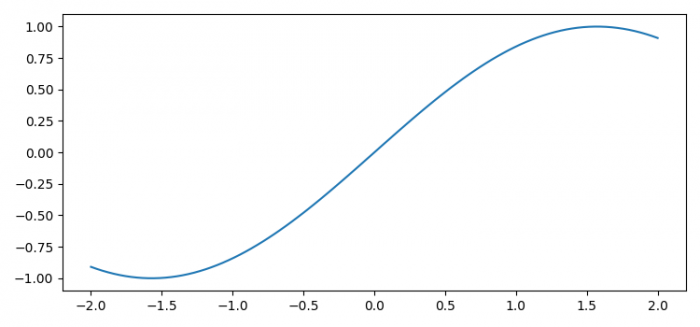# Setting the size of the plotting canvas in Matplotlib

To set the size of the plotting canvas in matplotlib, we can take the following steps:

• Set the figure size and adjust the padding between and around the subplots. Use figsize 7.50 and 3.50 to set the figure size.
• Create x and y data points using numpy.
• Plot x and y data points using plot() method.
• To display the figure, use show() method.

## Example

import numpy as np
from matplotlib import pyplot as plt
plt.rcParams["figure.figsize"] = [7.50, 3.50]
plt.rcParams["figure.autolayout"] = True
x = np.linspace(-2, 2, 100)
y = np.sin(x)
plt.plot(x, y)
plt.show()

## Output